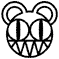Maths

(Redirected from Math)
Whoops(BOOM HEADSHOT)! did you mean Mathematics?

“How sex is related to math: "Add the right mood, subtract the clothes, divide the legs and mulitply."”

Proof that Testicles is Sexy:

If we accept that:

${\text{Testicles}}=({\text{Sex}}-{\text{Wrinkles}}){\text{Manliness}}''$We all know that Sex = 69, and that Wrinkles = Age. Testicles being 16 years old makes the equation looks like this:

${\text{Testicles}}=(69-16){\text{Man}}=53*{\text{Man}}''$Manliness is determined at birth by the sperm and the egg, sooooo:

${\text{Man}}={\text{XY}}''$Taking that into account the equation finally resembles this:

${\text{Testicles}}=53*{\text{XY}}=53{\text{XY}}$So, in conclusion Testicles is SEXY:

“Sir, are you sure there wasn't any algerithms in that drink?”

~ Student

“Who the hell are you?”

~ Drunken Maths Teacher

~ The "Mathematician" on how he PWN5 1337ly

“Sir, what are you doing under your desk with no clothes on?”

~ Student

“Mathe!”

~ Maths TeacherThis page has received the blessing of The Great Gozimnot of Mathematics, and is therefore mathematically correct.